### Introductory Examples

The following introductory examples illustrate how to get started using the NLP procedure.

#### An Unconstrained Problem

Consider the simple example of minimizing the Rosenbrock function (Rosenbrock 1960):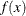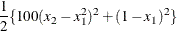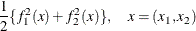The minimum function value is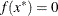at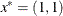. This problem does not have any constraints.

The following statements can be used to solve this problem:

proc nlp;
min f;
decvar x1 x2;
f1 = 10 * (x2 - x1 * x1);
f2 = 1 - x1;
f  = .5 * (f1 * f1 + f2 * f2);
run;


The MIN statement identifies the symbol f that characterizes the objective function in terms of f1 and f2, and the DECVAR statement names the decision variables x1 and x2. Because there is no explicit optimizing algorithm option specified (TECH=), PROC NLP uses the Newton-Raphson method with ridging, the default algorithm when there are no constraints.

A better way to solve this problem is to take advantage of the fact that
is a sum of squares of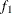and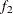and to treat it as a least squares problem. Using the LSQ statement instead of the MIN statement tells the procedure that this is a least squares problem, which results in the use of one of the specialized algorithms for solving least squares problems (for example, Levenberg-Marquardt).

proc nlp;
lsq f1 f2;
decvar x1 x2;
f1 = 10 * (x2 - x1 * x1);
f2 = 1 - x1;
run;


The LSQ statement results in the minimization of a function that is the sum of squares of functions that appear in the LSQ statement. The least squares specification is preferred because it enables the procedure to exploit the structure in the problem for numerical stability and performance.

PROC NLP displays the iteration history and the solution to this least squares problem as shown in Figure 7.1. It shows that the solution has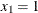and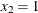. As expected in an unconstrained problem, the gradient at the solution is very close to.

Figure 7.1: Least Squares Minimization

PROC NLP: Least Squares Minimization

Levenberg-Marquardt Optimization

Scaling Update of More (1978)

 Parameter Estimates 2 2

Optimization Start
Active Constraints 0 Objective Function 0.7264362643

Iteration   Restarts Function
Calls
Active
Constraints
Objective
Function
Objective
Function
Change
Max Abs
Element
Lambda Ratio
Between
Actual
and
Predicted
Change
1   0 2 0   0.03373 0.6927 5.1950 0 0.954
2   0 3 0   1.5777E-30 0.0337 1.78E-15 0 1.000

Optimization Results
Iterations 2 Function Calls 4
Jacobian Calls 3 Active Constraints 0
Objective Function 1.577722E-30 Max Abs Gradient Element 1.776357E-15
Lambda 0 Actual Over Pred Change 1

 ABSGCONV convergence criterion satisfied.

PROC NLP: Least Squares Minimization

Optimization Results
Parameter Estimates
Objective
Function
1 x1 1.000000 1.776357E-15
2 x2 1.000000 0

Value of Objective Function = 1.577722E-30

#### Boundary Constraints on the Decision Variables

Bounds on the decision variables can be used. Suppose, for example, that it is necessary to constrain the decision variables in the previous example to be less than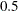. That can be done by adding a BOUNDS statement.

proc nlp;
lsq f1 f2;
decvar x1 x2;
bounds x1-x2 <= .5;
f1 = 10 * (x2 - x1 * x1);
f2 = 1 - x1;
run;


The solution in Figure 7.2 shows that the decision variables meet the constraint bounds.

Figure 7.2: Least Squares with Bounds Solution

PROC NLP: Least Squares Minimization

Value of Objective Function = 29.25

PROC NLP: Least Squares Minimization

Optimization Results
Parameter Estimates
Objective
Function
Active
Bound
Constraint
1 x1 0.500000 -0.500000 Upper BC
2 x2 0.250000 0

#### Linear Constraints on the Decision Variables

More general linear equality or inequality constraints of the form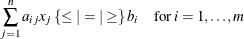can be specified in a LINCON statement. For example, suppose that in addition to the bounds constraints on the decision variables it is necessary to guarantee that the sum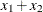is less than or equal to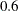. That can be achieved by adding a LINCON statement:

proc nlp;
lsq f1 f2;
decvar x1 x2;
bounds x1-x2 <= .5;
lincon x1 + x2 <= .6;
f1 = 10 * (x2 - x1 * x1);
f2 = 1 - x1;
run;


The output in Figure 7.3 displays the iteration history and the convergence criterion.

Figure 7.3: Least Squares with Bounds and Linear Constraints Iteration History

PROC NLP: Least Squares Minimization

Value of Objective Function = 29.25

PROC NLP: Least Squares Minimization

Levenberg-Marquardt Optimization

 Parameter Estimates 2 2 0 2 1

Optimization Start
Active Constraints 0 Objective Function 29.25

Iteration   Restarts Function
Calls
Active
Constraints
Objective
Function
Objective
Function
Change
Max Abs
Element
Lambda Ratio
Between
Actual
and
Predicted
Change
1   0 3 0   8.19877 21.0512 39.5420 0.0170 0.729
2   0 4 0   1.05752 7.1412 13.6170 0.0105 0.885
3   0 5 1   1.04396 0.0136 18.6337 0 0.0128
4   0 6 1   0.16747 0.8765 0.5552 0 0.997
5   0 7 1   0.16658 0.000895 0.000324 0 0.998
6   0 8 1   0.16658 3.06E-10 5.911E-7 0 0.998

Optimization Results
Iterations 6 Function Calls 9
Jacobian Calls 7 Active Constraints 1
Objective Function 0.1665792899 Max Abs Gradient Element 5.9108825E-7
Lambda 0 Actual Over Pred Change 0.998176801

 GCONV convergence criterion satisfied.

Figure 7.4 shows that the solution satisfies the linear constraint. Note that the procedure displays the active constraints (the constraints that are tight) at optimality.

Figure 7.4: Least Squares with Bounds and Linear Constraints Solution

PROC NLP: Least Squares Minimization

Scaling Update of More (1978)

PROC NLP: Least Squares Minimization

Optimization Results
Parameter Estimates
Objective
Function
1 x1 0.423645 -0.312000
2 x2 0.176355 -0.312001

Linear Constraints Evaluated at Solution
1 ACT 0 = 0.6000 - 1.0000 * x1 - 1.0000 * x2

#### Nonlinear Constraints on the Decision Variables

More general nonlinear equality or inequality constraints can be specified using an NLINCON statement. Consider the least squares problem with the additional constraint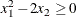This constraint is specified by a new function c1 constrained to be greater than or equal to 0 in the NLINCON statement. The function c1 is defined in the programming statements.

proc nlp tech=QUANEW;
min f;
decvar x1 x2;
bounds x1-x2 <= .5;
lincon x1 + x2 <= .6;
nlincon c1 >= 0;

c1 = x1 * x1 - 2 * x2;

f1 = 10 * (x2 - x1 * x1);
f2 = 1 - x1;

f = .5 * (f1 * f1 + f2 * f2);
run;


Figure 7.5 shows the iteration history, and Figure 7.6 shows the solution to this problem.

Figure 7.5: Least Squares with Bounds, Linear and Nonlinear Constraints, Iteration History

PROC NLP: Nonlinear Minimization

Dual Quasi-Newton Optimization

Modified VMCWD Algorithm of Powell (1978, 1982)

Dual Broyden - Fletcher - Goldfarb - Shanno Update (DBFGS)

Lagrange Multiplier Update of Powell(1982)

 Parameter Estimates 2 0 2 1 1

Optimization Start
Objective Function 29.25 Maximum Constraint Violation 0
Maximum Gradient of the Lagran Func 76.5

Iteration   Restarts Function
Calls
Objective
Function
Maximum
Constraint
Violation
Predicted
Function
Reduction
Step
Size
Maximum
Element
of the
Lagrange
Function
1   0 4 2.88501 0 2.9362 1.000 20.961
2   0 5 0.91110 0 0.5601 1.000 6.777
3   0 6 0.61803 0 0.00743 1.000 1.148
4 ' 0 7 0.61090 0 0.0709 1.000 1.194
5 ' 0 8 0.54427 0 0.6015 1.000 0.988
6   0 10 0.49223 0 0.3369 0.100 0.970
7   0 12 0.45729 0 0.1848 0.114 1.332
8   0 14 0.40786 0 0.0749 0.355 2.390
9   0 15 0.36176 0 0.0556 1.000 1.129
10   0 16 0.33086 0 0.00178 1.000 0.139
11   0 17 0.33017 0 0.000290 1.000 0.0521
12   0 18 0.33004 0 0.000012 1.000 0.00222
13   0 19 0.33003 0 2.963E-8 1.000 0.00004

Optimization Results
Iterations 13 Function Calls 20
Gradient Calls 16 Active Constraints 1
Objective Function 0.3300307304 Maximum Constraint Violation 0
Maximum Projected Gradient 0.0000142688 Value Lagrange Function 0.3300307155
Maximum Gradient of the Lagran Func 0.0000138527 Slope of Search Direction -2.962973E-8

Figure 7.6: Least Squares with Bounds, Linear and Nonlinear Constraints, Solution

PROC NLP: Nonlinear Minimization

Optimization Results
Parameter Estimates
Objective
Function
Lagrange
Function
1 x1 0.246953 0.753007 -0.000019007
2 x2 0.030493 -3.049279 -0.000004694

Value of Objective Function = 0.3300307281

Value of Lagrange Function = 0.3300307156

Linear Constraints Evaluated at Solution
1   0.32255 = 0.6000 - 1.0000 * x1 - 1.0000 * x2

Values of Nonlinear Constraints
Constraint Value Residual Lagrange
Multiplier

[ 2 ] c1_G 8.244E-9 8.244E-9 1.5246 Active NLIC

Not all of the optimization methods support nonlinear constraints. In particular the Levenberg-Marquardt method, the default for LSQ, does not support nonlinear constraints. (For more information about the particular algorithms, see the section Optimization Algorithms.) The Quasi-Newton method is the prime choice for solving nonlinear programs with nonlinear constraints. The option TECH=QUANEW in the PROC NLP statement causes the Quasi-Newton method to be used.

#### A Simple Maximum Likelihood Example

The following is a very simple example of a maximum likelihood estimation problem with the log likelihood function: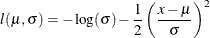The maximum likelihood estimates of the parametersandform the solution to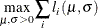where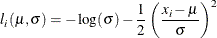In the following DATA step, values forare input into SAS data set X; this data set provides the values of.

data x;
input x @@;
datalines;
1 3 4 5 7
;


In the following statements, the DATA=X specification drives the building of the objective function. When each observation in the DATA=X data set is read, a new term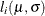using the value ofis added to the objective function LOGLIK specified in the MAX statement.

proc nlp data=x vardef=n covariance=h pcov phes;
profile mean sigma / alpha=.5 .1 .05 .01;
max loglik;
parms mean=0, sigma=1;
bounds sigma > 1e-12;
loglik=-0.5*((x-mean)/sigma)**2-log(sigma);
run;


After a few iterations of the default Newton-Raphson optimization algorithm, PROC NLP produces the results shown in Figure 7.7.

Figure 7.7: Maximum Likelihood Estimates

PROC NLP: Nonlinear Maximization

Optimization Results
Parameter Estimates
N Parameter Estimate Approx
Std Err
t Value Approx
Pr > |t|
Objective
Function
1 mean 4.000000 0.894427 4.472136 0.006566 -1.33149E-10
2 sigma 2.000000 0.632456 3.162278 0.025031 5.6064146E-9

Value of Objective Function = -5.965735903

In unconstrained maximization, the gradient (that is, the vector of first derivatives) at the solution must be very close to zero and the Hessian matrix at the solution (that is, the matrix of second derivatives) must have nonpositive eigenvalues. The Hessian matrix is displayed in Figure 7.8.

Figure 7.8: Hessian Matrix

PROC NLP: Nonlinear Maximization

Hessian Matrix
mean sigma
mean -1.250000003 1.33149E-10
sigma 1.33149E-10 -2.500000014

Determinant = 3.1250000245

Matrix has Only Negative Eigenvalues

Under reasonable assumptions, the approximate standard errors of the estimates are the square roots of the diagonal elements of the covariance matrix of the parameter estimates, which (because of the COV=H specification) is the same as the inverse of the Hessian matrix. The covariance matrix is shown in Figure 7.9.

Figure 7.9: Covariance Matrix

PROC NLP: Nonlinear Maximization

Covariance Matrix 2: H = (NOBS/d)
inv(G)
mean sigma
mean 0.7999999982 4.260769E-11
sigma 4.260769E-11 0.3999999978

Factor sigm = 1

Determinant = 0.3199999975

Matrix has 2 Positive Eigenvalue(s)

The PROFILE statement computes the values of the profile likelihood confidence limits on SIGMA and MEAN, as shown in Figure 7.10.

Figure 7.10: Confidence Limits

PROC NLP: Nonlinear Maximization

Wald and PL Confidence Limits
N Parameter Estimate Alpha Profile Likelihood Confidence
Limits
Wald Confidence Limits
1 mean 4.000000 0.500000 3.384431 4.615569 3.396718 4.603282
1 mean . 0.100000 2.305716 5.694284 2.528798 5.471202
1 mean . 0.050000 1.849538 6.150462 2.246955 5.753045
1 mean . 0.010000 0.670351 7.329649 1.696108 6.303892
2 sigma 2.000000 0.500000 1.638972 2.516078 1.573415 2.426585
2 sigma . 0.100000 1.283506 3.748633 0.959703 3.040297
2 sigma . 0.050000 1.195936 4.358321 0.760410 3.239590
2 sigma . 0.010000 1.052584 6.064107 0.370903 3.629097